Open in App
Not now

# Ratio of area of two nested polygons formed by connecting midpoints of sides of a regular N-sided polygon

• Last Updated : 17 May, 2021

Given an N-sided polygon, the task is to find the ratio of the area of the Nth to (N + 1)th N-sided regular nested polygons generated by joining the midpoints of the sides of the original polygon.

Examples :

Input: N = 3
Output: 4.000000
Explanation: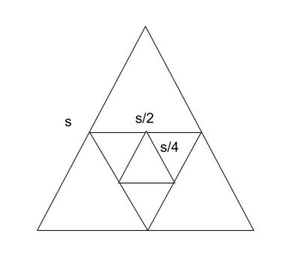Nested Triangle

Ratio of the length of the sides formed by joining the mid-points of the triangle with the length of the side of the original triangle is 0.5. Hence, R = (Area of Nth triangle) / (Area of (N + 1)th triangle) = 4

Input: N = 4
Output: 2.000000

Approach: The problem can be solved based on the following observations:

• Consider an N-sided regular polygon as shown in the figure below.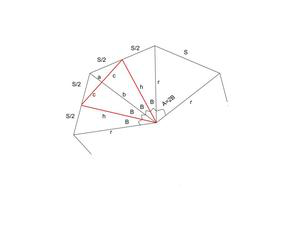Representation Of Nested regular polygon of N sides.

• A = 2 * ℼ / N
B = ℼ / N
h = r * cos(B)
b = h * cos(B)
c = h((1 – cos(A)) / 2)1/2
• Area of the Black Isosceles Triangle: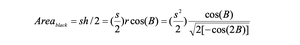• Area of the Red Isosceles Triangle: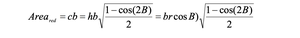• r = s / (2 * [1 – cos(2B)])1/2 and b = r * [cos(B)]2
• After combining the above equations: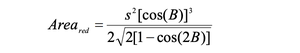• Final result obtained is as follows: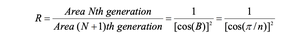Below is the implementation of the above approach:

## C++

 `// C++ code for the above approach` `#include ` `using` `namespace` `std;`   `// Function to calculate the ratio of` `// area of N-th and (N + 1)-th nested` `// polygons formed by connecting midpoints` `void` `AreaFactor(``int` `n)` `{` `    ``// Stores the value of PI` `    ``double` `pi = 3.14159265;`   `    ``// Calculating area the factor` `    ``double` `areaf = 1 / (``cos``(pi / n)` `                        ``* ``cos``(pi / n));`   `    ``// Printing the ratio` `    ``// precise upto 6 decimal places` `    ``cout << fixed << setprecision(6)` `         ``<< areaf << endl;` `}`   `// Driver Code` `int` `main()` `{` `    ``int` `n = 4;` `    ``AreaFactor(n);`   `    ``return` `0;` `}`

## Java

 `// Java program for the above approach` `import` `java.io.*;`   `class` `GFG{`   `// Function to calculate the ratio of` `// area of N-th and (N + 1)-th nested` `// polygons formed by connecting midpoints` `static` `void` `AreaFactor(``int` `n)` `{` `    `  `    ``// Stores the value of PI` `    ``double` `pi = ``3.14159265``;` ` `  `    ``// Calculating area the factor` `    ``double` `areaf = ``1` `/ (Math.cos(pi / n) * ` `                        ``Math.cos(pi / n));` ` `  `    ``// Printing the ratio` `    ``// precise upto 6 decimal places` `    ``System.out.format(``"%.6f"``, areaf);` `}`   `// Driver Code` `public` `static` `void` `main(String[] args)` `{` `    ``int` `n = ``4``;` `    `  `    ``AreaFactor(n);` `}` `}`   `// This code is contributed by code_hunt`

## Python3

 `# Python3 code for the above approach` `import` `math`   `# Function to calculate the ratio of` `# area of N-th and (N + 1)-th nested` `# polygons formed by connecting midpoints` `def` `AreaFactor(n):`   `    ``# Stores the value of PI` `    ``pi ``=` `3.14159265`   `    ``# Calculating area the factor` `    ``areaf ``=` `1` `/` `(math.cos(pi ``/` `n) ``*` `                 ``math.cos(pi ``/` `n))`   `    ``# Printing the ratio` `    ``# precise upto 6 decimal places` `    ``print``(``'%.6f'` `%` `areaf)` `    `  `# Driver Code` `if` `__name__ ``=``=` `"__main__"``:` `  `  `    ``n ``=` `4` `    ``AreaFactor(n)`   `# This code is contributed by ukasp`

## C#

 `// C# program for the above approach` `using` `System;` `using` `System.Collections.Generic;`   `class` `GFG {`   `// Function to calculate the ratio of` `// area of N-th and (N + 1)-th nested` `// polygons formed by connecting midpoints` `static` `void` `AreaFactor(``int` `n)` `{` `     `  `    ``// Stores the value of PI` `    ``double` `pi = 3.14159265;` `  `  `    ``// Calculating area the factor` `    ``double` `areaf = 1 / (Math.Cos(pi / n) *` `                        ``Math.Cos(pi / n));` `  `  `    ``// Printing the ratio` `    ``// precise upto 6 decimal places` `    ``Console.WriteLine(Math.Round(areaf));`   `}` `    ``// Driver Code` `    ``public` `static` `void` `Main(``string``[] args)` `    ``{` `        ``int` `n = 4;` `     `  `        ``AreaFactor(n);` `    ``}` `}`   `// This code is contributed by susmitakundugoaldanga.`

## Javascript

 ``

Output:

`2.000000`

Time Complexity: O(1)
Auxiliary Space: O(1)

My Personal Notes arrow_drop_up
Related Articles